# Converting Transfer Function Into Circuits

#### aarontan0219

Joined Nov 16, 2019
22
I am given a transfer function in Modern Control system and are asked to form a real circuit respect to the transfer function as well as the characteristics such as rise time, etc. The transfer function are given as $\frac{V_{out} (s)}{V_{in} (s)} = \frac{198025}{s^2+455s+198025}$ . I dont really understand this tocpic and hope to het help and guiding me to solve this question. Really need help in this assignment as my coursework marks are in RED color. It is due on 24/11/2019. Hope it really gets help on solving this problem. Thanks!

#### Jony130

Joined Feb 17, 2009
5,445

#### aarontan0219

Joined Nov 16, 2019
22
Is that the response due to the transfer function? I had simulated those in MATlab but I wondering what circuits can form the plot.
The requirements need for the circuit is being formed by integrator circuit, unity gain inverter circuits , summing amplifier, non inverting amplifier and/or combinations from them.

#### Jony130

Joined Feb 17, 2009
5,445
As you can see from the Bode plot your job is to build a second-order low-pass filter. Use the transfer function to find the pole locations and choose the filter topology and solve for component values based on pole locations.

#### Papabravo

Joined Feb 24, 2006
20,154
The differential equations which describe an RLC circuit and a spring-mass-damper system have the same form. Once you have analyzed either system you can formulate either a circuit or a mechanical system that will behave according to the differential equation.

#### MrAl

Joined Jun 17, 2014
10,091
I am given a transfer function in Modern Control system and are asked to form a real circuit respect to the transfer function as well as the characteristics such as rise time, etc. The transfer function are given as $\frac{V_{out} (s)}{V_{in} (s)} = \frac{198025}{s^2+455s+198025}$ . I dont really understand this tocpic and hope to het help and guiding me to solve this question. Really need help in this assignment as my coursework marks are in RED color. It is due on 24/11/2019. Hope it really gets help on solving this problem. Thanks!

Hi there,

If you have no other constrains on how to implement this then the simplest way is purely algebraic where we cross multiply and then separate into individual integrators. You can then use op amp integrators for the implementation.

This is so easy to do i almost hate to mention it :--)
But if you need more help that's ok it might be your first time doing this.

Here's a quick example....

System:
Vout/Vin=(s+1)/(s^2+2*s+3)

Cross multiply and get:
(s^2+2*s+3)*Vout=(s+1)*Vin

Expand and get:
s^2*Vout+2*s*Vout+3*Vout=s*Vin+Vin

Divide by highest power of 's' and get:
(s^2*Vout+2*s*Vout+3*Vout)/s^2=(s*Vin+Vin)/s^2

Expand that and get:
(2*Vout)/s+(3*Vout)/s^2+Vout=Vin/s+Vin/s^2

Solve that for the lone "Vout" term and get:
Vout=-2*Vout/s-3*Vout/s^2+Vin/s+Vin/s^2

That is the final math form which is sort of canonical in that we have Vout alone on the left and various terms with both Vin and Vout on the right and divisions involving powers of 's' as well as constant gains (-2, -3, and 1).
The interpretation of this final form is that anything on the right side with Vin in it is in the forward path and anything on the right side with Vout in it is in the feedback path, and any power of 's' represents an integration where the number of cascaded integrators is equal to that power of 's'.
So Vin gets integrated twice and so does Vout and the terms with Vout are negative so there is also an amplification by minus 2 or minus 3. The circuit therefore has two integrators in it and possibly some amplifiers that implement the gains -2 and -3. There are various ways to implement the gains (such as combining them) although that's the most straightforward.

Now try this on your system.

The attachment is a comparison of the step response of the example system, first a simulation after implementation as above and then as the time function found from the Inverse Laplace Transform.

Last edited:

#### aarontan0219

Joined Nov 16, 2019
22
As you can see from the Bode plot your job is to build a second-order low-pass filter. Use the transfer function to find the pole locations and choose the filter topology and solve for component values based on pole locations.
Ok ill try it out thanks

#### aarontan0219

Joined Nov 16, 2019
22
The differential equations which describe an RLC circuit and a spring-mass-damper system have the same form. Once you have analyzed either system you can formulate either a circuit or a mechanical system that will behave according to the differential equation.
Noted thanks will try it out myself

#### aarontan0219

Joined Nov 16, 2019
22
Hi there,

If you have no other constrains on how to implement this then the simplest way is purely algebraic where we cross multiply and then separate into individual integrators. You can then use op amp integrators for the implementation.

This is so easy to do i almost hate to mention it :--)
But if you need more help that's ok it might be your first time doing this.

Here's a quick example....

System:
Vout/Vin=(s+1)/(s^2+2*s+3)

Cross multiply and get:
(s^2+2*s+3)*Vout=(s+1)*Vin

Expand and get:
s^2*Vout+2*s*Vout+3*Vout=s*Vin+Vin

Divide by highest power of 's' and get:
(s^2*Vout+2*s*Vout+3*Vout)/s^2=(s*Vin+Vin)/s^2

Expand that and get:
(2*Vout)/s+(3*Vout)/s^2+Vout=Vin/s+Vin/s^2

Solve that for the lone "Vout" term and get:
Vout=-2*Vout/s-3*Vout/s^2+Vin/s+Vin/s^2

That is the final math form which is sort of canonical in that we have Vout alone on the left and various terms with both Vin and Vout on the right and divisions involving powers of 's' as well as constant gains (-2, -3, and 1).
The interpretation of this final form is that anything on the right side with Vin in it is in the forward path and anything on the right side with Vout in it is in the feedback path, and any power of 's' represents an integration where the number of cascaded integrators is equal to that power of 's'.
So Vin gets integrated twice and so does Vout and the terms with Vout are negative so there is also an amplification by minus 2 or minus 3. The circuit therefore has two integrators in it and possibly some amplifiers that implement the gains -2 and -3. There are various ways to implement the gains (such as combining them) although that's the most straightforward.

Now try this on your system.

The attachment is a comparison of the step response of the example system, first a simulation after implementation as above and then as the time function found from the Inverse Laplace Transform.
Sorry for letting you explain these simple things for my questions. I'm not good in this subject. Thanks for the detail explaination. I will try it out and see weather it works. Thanks!

#### MrAl

Joined Jun 17, 2014
10,091
Hello again,

Oh it works but what i left out for now is how to get from the block diagram to the actual circuit with resistors and capacitors and op amps.
The information i gave you gets you as far as the block diagram and from there you have to figure out a circuit to perform the integrations and set the gains. If you have trouble there too we can go over an example in full including going from block diagram to actual circuit. That is where the implementation becomes very variable with a lot of different ways to do it.

#### aarontan0219

Joined Nov 16, 2019
22
Hello again,

Oh it works but what i left out for now is how to get from the block diagram to the actual circuit with resistors and capacitors and op amps.
The information i gave you gets you as far as the block diagram and from there you have to figure out a circuit to perform the integrations and set the gains. If you have trouble there too we can go over an example in full including going from block diagram to actual circuit. That is where the implementation becomes very variable with a lot of different ways to do it.
I had tried it and get the circuit but when i simulate it with LT Spice with the designed circuit, I could not get the desired output. I have attached my calculation and design circuit as well as the simulated output due to step response and also the expected graph should be get by the transfer function given.
Another question is, any external circuit could be added before the circuit to overcome the steady state error?

This is my calcualtion (sorry that I miss written the 445 as 455 but already corrected it over my calculation for value)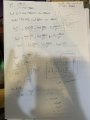This is my circuit based on the design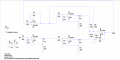Response of the circuit to step response ( it suppose to be going back to 1V after transient state but it ends up growing to infinite)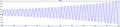Expected result from MATLab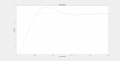Below is my attachment circuit diagram done by LT Spice

#### Attachments

• 4.2 KB Views: 9

#### LvW

Joined Jun 13, 2013
1,647
I am given a transfer function in Modern Control system and are asked to form a real circuit respect to the transfer function as well as the characteristics such as rise time, etc. The transfer function are given as $\frac{V_{out} (s)}{V_{in} (s)} = \frac{198025}{s^2+455s+198025}$ . I dont really understand this tocpic and hope to het help and guiding me to solve this question. Really need help in this assignment as my coursework marks are in RED color. It is due on 24/11/2019. Hope it really gets help on solving this problem. Thanks!
Aaron - this is nothing else than a simple 2nd-oder lowpass function which can be realized with one single opamp and 6 passive elements....(as a maximum). I cannot understand why you think that 4 integrators are necessary. It is a second-order function only!!
Question: Do you know how the general second-order lowpass function looks like? Then, you have nothing to do than to to compare the respective expressions (s², s, const).
As an alternative, you can find the roots of the denominator (poles of the transfer function) and use pole tables for finding the parts values.

Last edited:

#### MrAl

Joined Jun 17, 2014
10,091
I had tried it and get the circuit but when i simulate it with LT Spice with the designed circuit, I could not get the desired output. I have attached my calculation and design circuit as well as the simulated output due to step response and also the expected graph should be get by the transfer function given.
Another question is, any external circuit could be added before the circuit to overcome the steady state error?

This is my calcualtion (sorry that I miss written the 445 as 455 but already corrected it over my calculation for value)
View attachment 191911

This is my circuit based on the design
View attachment 191912

Response of the circuit to step response ( it suppose to be going back to 1V after transient state but it ends up growing to infinite)
View attachment 191913

Expected result from MATLab
View attachment 191914

Below is my attachment circuit diagram done by LT Spice

Hello again,

I think you have the basic idea but it is a little too over complicated in that there are too many integrations (i count 6 in total). You only need two integrators for the entire circuit as a block diagram and actual circuit also.

Also, what are you expecting from the RC combinations such as R4 and C4?
You know that is not a pure integrator right? You may be able to fit one of those sections into the circuit once you get the main concept (such as with a block diagram) but it would be best to start out simple by starting with two non inverting integrators and work your way into a real circuit.

So what you should do is start with two non inverting integrators and try to draw up the block diagram. I can do that for you but you should really try to think about this yourself first. To start, think of two non inverting integrators in cascade and what you need to do to modify that circuit to get all the forward and feedback paths included.
Note that you will need summing blocks and if you dont use inverters for the two gains that are negative you will also need two subtractor blocks.

You may even want to start with a signal flow graph, but a block diagram works just as well. Once you get either of these the circuit will be easier to visualize.
So the technique is:
1. Start with non inverting integrators in cascade, the number of integrators equals the order of the system.
2. Create a block diagram using that above.
3. Figure out what real components will be necessary to implement the function of the block diagram.

Step 1 is quite straightforward.
Step 2 involves some thought as to how to place the idealized forward and feedback paths and gains.
Step 3 can be done a lot of different ways, we can go over some of those here. This involves op amp(s), resistors, and capacitors, and some idea about what is practical for any circuit.

I can promise you that if you follow these three steps (after the math) you will be able to do any system no matter how complicated almost as easy as this one.

Last edited:

#### MrAl

Joined Jun 17, 2014
10,091
Here is an example to help.

#### aarontan0219

Joined Nov 16, 2019
22
Hello again,

I think you have the basic idea but it is a little too over complicated in that there are too many integrations (i count 6 in total). You only need two integrators for the entire circuit as a block diagram and actual circuit also.

Also, what are you expecting from the RC combinations such as R4 and C4?
You know that is not a pure integrator right? You may be able to fit one of those sections into the circuit once you get the main concept (such as with a block diagram) but it would be best to start out simple by starting with two non inverting integrators and work your way into a real circuit.

So what you should do is start with two non inverting integrators and try to draw up the block diagram. I can do that for you but you should really try to think about this yourself first. To start, think of two non inverting integrators in cascade and what you need to do to modify that circuit to get all the forward and feedback paths included.
Note that you will need summing blocks and if you dont use inverters for the two gains that are negative you will also need two subtractor blocks.

You may even want to start with a signal flow graph, but a block diagram works just as well. Once you get either of these the circuit will be easier to visualize.
So the technique is:
1. Start with non inverting integrators in cascade, the number of integrators equals the order of the system.
2. Create a block diagram using that above.
3. Figure out what real components will be necessary to implement the function of the block diagram.

Step 1 is quite straightforward.
Step 2 involves some thought as to how to place the idealized forward and feedback paths and gains.
Step 3 can be done a lot of different ways, we can go over some of those here. This involves op amp(s), resistors, and capacitors, and some idea about what is practical for any circuit.

I can promise you that if you follow these three steps (after the math) you will be able to do any system no matter how complicated almost as easy as this one.
The R4 and C4 is a part of the non inverting integrator circuit which is same for the bottom 2 circuit. My circuit is made from 1 inverting and 4 non inverting. The image below is the non inverting integrator i searched. Is it correct? If not please let me know the correct one.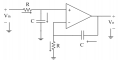I started with with your steps and this is the circuit i made. Is it correct?
The U1 and U2 are two non inverting integrator, U4 and U5 are inverting amplifier to invert the output of U1 and output of cascaded U1 and U2 to fulfill my equation. U3 is a non inverting summing amplifier to sum all the 3 input to get the final output. But in this case, i no longer have (Vin*198025)/^2 as it is now feedback by the output. If can please give me the solution as Im in a hurry now. The deadline is this coming Friday and I need to build up the circuit with component and test the performance in real life.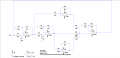#### LvW

Joined Jun 13, 2013
1,647
Hi Aaaron - OK, if you like - you can create a second-order lowpass on the basis of integrating circuits. However, why do you stick on non-inv. interators?
As an example, I recommend to look at the structure of a well-known 2nd-order filter called "KHN Filter" (you can google it). It is based on two simple inverting (MILLER) integrators and one additional opamp for superimposing the feedback signals.

#### aarontan0219

Joined Nov 16, 2019
22
Hi Aaaron - OK, if you like - you can create a second-order lowpass on the basis of integrating circuits. However, why do you stick on non-inv. interators?
As an example, I recommend to look at the structure of a well-known 2nd-order filter called "KHN Filter" (you can google it). It is based on two simple inverting (MILLER) integrators and one additional opamp for superimposing the feedback signals.
Is it something like this? Then is the value of R and C same as what i calculated on top?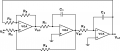#### LvW

Joined Jun 13, 2013
1,647
Yes - that is one of the classical integrator filter topology (KHN structure).
The pole frequency is wp=SQRT(1/kr*T1*T2) with kr=R5/R6 and T1=R1C1 and T2=R2C2

Last edited:

#### aarontan0219

Joined Nov 16, 2019
22
Yes - that is one of the classical integrator filter topology (KHN structure).
The pole frequency is wp=SQRT(1/kr*T1*T2) with kr=R5/R6 and T1=R1C1 and T2=R2C2
So to fulfil my requirement, all my R value will be 1000 ohm and all my C value will be 2.2uF right? Then what does the R3 and R4 does? What will be the overall transfer function?

#### MrAl

Joined Jun 17, 2014
10,091
The R4 and C4 is a part of the non inverting integrator circuit which is same for the bottom 2 circuit. My circuit is made from 1 inverting and 4 non inverting. The image below is the non inverting integrator i searched. Is it correct? If not please let me know the correct one.View attachment 191977

I started with with your steps and this is the circuit i made. Is it correct?
The U1 and U2 are two non inverting integrator, U4 and U5 are inverting amplifier to invert the output of U1 and output of cascaded U1 and U2 to fulfill my equation. U3 is a non inverting summing amplifier to sum all the 3 input to get the final output. But in this case, i no longer have (Vin*198025)/^2 as it is now feedback by the output. If can please give me the solution as Im in a hurry now. The deadline is this coming Friday and I need to build up the circuit with component and test the performance in real life.
View attachment 191979
Hello,

You still have too many integrations so it cant be right base on just that fact alone.
Also, the signal R and C "integrators" are not true integrators. They are low pass filters which are not just integrators even though they contain an integrator.

You need to create a block diagram first. That't the general technique. If you take shortcuts you wont learn the general way to do these so when something more complicated comes up in the future you wont know what to do.